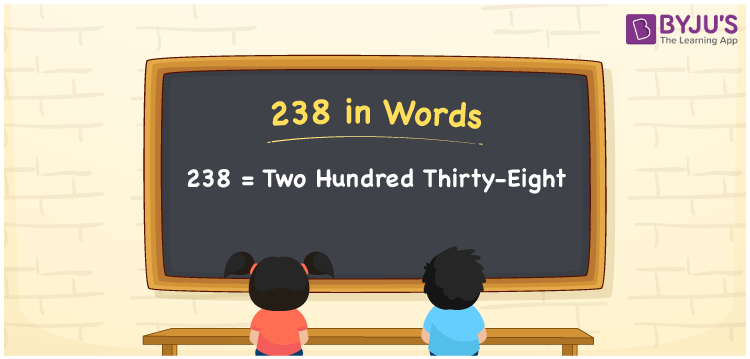# 238 in Words

We can write 238 in words as Two hundred thirty-eight which is called the number name or word form of 238. Suppose you purchased a kg of apples at Rs. 238, then you can say, “I purchased a kg of apples for Two hundred thirty-eight rupees”. Also, it is known that 238 is a cardinal number since it represents a specific amount or quantity.

 238 in words Two hundred thirty-eight Two hundred thirty-eight in Numbers 238

## 238 in English Words

We generally write numbers in words using the English alphabet. So, we can spell 238 in English words as “Two hundred thirty-eight”.## How to Write 238 in Words?

Let’s learn how to write the number 238 in words using a place value chart given here.

 Hundreds Tens Ones 2 3 8

Here, ones = 8, tens = 3, hundreds = 2

These numbers can be written as:

2 × Hundred + 3 × Ten + 8 × One

= 2 × 100 + 3 × 10 + 8 × 1

= 200 + 30 + 8

= Two hundred + Thirty + Eight

= Two hundred thirty-eight

Therefore, 238 in words = Two hundred thirty-eight

238 is a natural number that precedes 239 and succeeds 237.

238 in words – Two hundred thirty-eight

Is 238 an odd number? – No

Is 238 an even number? – Yes

Is 238 a prime number? – No

Is 238 a composite number? – Yes

Is 238 a perfect square number? – No

Is 238 a perfect cube number? – No

## Frequently Asked Questions on 238 in Words

Q1

### How do you write 238 in words?

The number 238 can be written in words as Two hundred thirty-eight.
Q2

### How to write Rs. 238 in words on a cheque?

On a cheque, we generally write Rs. 238 in words as “Two hundred thirty-eight rupees only”.
Q3

### What is 238 in word form?

Two hundred thirty-eight is 238 in word form.